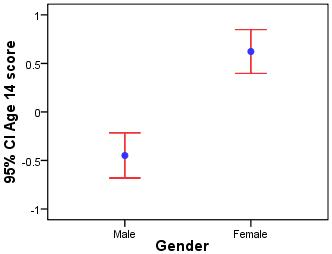# Using Statistical Regression Methods in Education Research# Glossary

 A B C D E F G H I J K L M N O P Q R S T U V W X Y Z

#### E

Effect size

The effect size is a standardized measure of the strength of an observed effect. It allows the researcher to gauge the magnitude of a relationship or difference on a standardized scale. There are a number of different measures of effect size including Cohen's d and Pearson's correlation coefficient.

Error Bars

Error bars display the means for different observations or categories along with the confidence intervals for those means (usually 95%). We have provided an example below (the mean is the blue dot with the red lines encompassing the 95% confidence interval for each group).Explanatory variable

Explanatory variables, sometimes called independent or predictor variables, are factors that are operationalised (made measurable) and used in regression to predict a given outcome. Explanatory variables can be almost anything you can think of that may be relevant to the outcome, though they need to be quantified sensibly if they are to be used in regression analysis.

Exponent

In basic terms, the exponent is the value you get if you take the inverse of the log function. For example, if you take the log of the odds then you get the log odds, if you took the exponent of the log odds you would get the odds.

Page contact: Feedback to ReStore team Last revised: Thu 28 Jul 2011